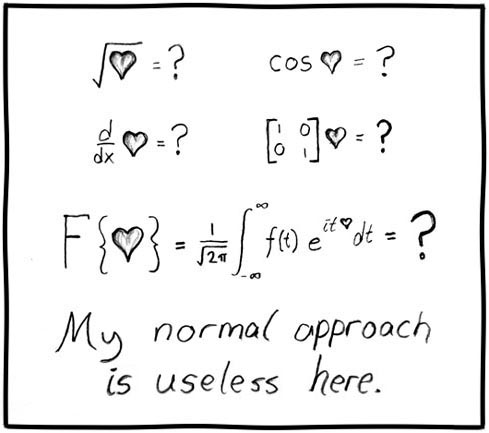← Back

January 27, 2006

# #55: Useless[[Different mathematic equations, all with heart on left side, and all equal question mark. Equations are as follows:

Square root of heart equals question mark

Cosinus of heart equals question mark

Derivative of heart with respect to x equals question mark

Identity matrix of heart equals question mark

Fourier transform of heart equals question mark]]

My normal approach is useless here